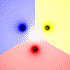27
Oct
07

### Magnetic Pendulum Strange AttractorThis chaotic strange attractor represent the final resting positions for a magnetic pendulum suspended over some magnets (shown as black dots). It kind of looks like mixed paint. The 2D animation shows what happens as you decrease the damping factor. The 3D animation was shaded by path length.

Mathematica 4.2 version: 1/24/05; C++ version: 10/27/07; 3D rendered in POV-Ray 3.1

```(* runtime: 25 seconds, increase n for higher resolution *) n = 40; h = 0.25; g = 0.2; mu = 0.07; zlist = {Sqrt + I, -Sqrt + I, -2I}; image = Table[z2 = z /. NDSolve[{z''[t] == Plus @@ ((zlist - z[t])/(h^2 + Abs[zlist - z[t]]^2)^1.5) - g z[t] - mu z'[t], z == x + I y, z' == 0}, z, {t, 0, 25}, MaxSteps -> 200000][]; r = Abs[z2 - zlist]; i = Position[r, Min[r]][[1, 1]]; Hue[i/3], {y, -5.0, 5.0, 10.0/n}, {x, -5.0,5.0, 10.0/n}]; Show[Graphics[RasterArray[image]], AspectRatio -> 1];```The picture on the left shows another version with five magnets. See also my physics pendulums.

Here is some Mathematica code to numerically solve this using the Beeman integration scheme with the predictor-corrector modification:
```(* runtime: 45 seconds, increase n for higher resolution *) n = 40; tmax = 25; dt = 0.1; h = 0.25; g = 0.2; mu = 0.07; zlist = {Sqrt + I, -Sqrt + I, -2I}; image = Table[z = x + I y; v = a = a1 = 0; Do[z += v dt + (4a - a1)dt^2/6; vpredict = v + (3a - a1)dt/2; a2 = Plus @@ ((zlist - z)/(h^2 + Abs[zlist - z]^2)^1.5) - g z - mu vpredict; v += (2a2 + 5a - a1)dt/6; a1 =a; a = a2, {t, 0, tmax, dt}]; r = Abs[z - zlist]; Hue[Position[r, Min[r]][[1, 1]]/3], {y, -5.0, 5.0, 10.0/n}, {x, -5.0, 5.0, 10.0/n}]; Show[Graphics[RasterArray[image]], AspectRatio -> 1];```

Advertisements

#### 4 Responses to “Magnetic Pendulum Strange Attractor”

1.1 Ingo Berg
April 1, 2009 at 8:31 pm

Hi Paul, nice to see you have opened your own blog. I’m always impressed when i see your work. As an addition i’d like to point all interested readers to a youtube video that explains the magnetic pendulum simulation very nicely: http://www.youtube.com/watch?v=Qe5Enm96MFQ

Regards,
Ingo

2.2 michael brandt
August 1, 2010 at 3:50 pm

my comment it all looks realy interesting but what is it all for is there any use behind your
logig or are the colours the important part for you:if you like science then take a peak at
my foto albums on face book science is also my favourite:stay healthy and prosper:

•3 Daan
June 16, 2015 at 8:19 am

Beautiful! Thanks for the code. I am making a real life magnetic pendulum right now. I have also adapted the code to run under a recent version of Mathematica. I have adapted it to produce increasing levels of zoom. I was expecting to find new patterns at every increased zoom level, like you would in a fractal. This would imply that no matter how precise you determine initial conditions, it will never be precise enough to determine the outcome. However, this is not what I found.

Why?

Here’s the code. Varying the “max” would determine where you are zooming in the positive quadrant.

n=30; h=0.25; g=0.2; mu=0.07; zlist={Sqrt+I,-Sqrt+I,-2I};
image[xmin_,xmax_,ymin_,ymax_] :=
ParallelTable[z2=z/.NDSolve[{z”[t]==Plus@@((zlist-z[t])/(h^2+Abs[zlist-z[t]]^2)^1.5)-g z[t]-mu z'[t],z==x+I y,z'==0},z,{t,0,25},MaxSteps->20000][];
r=Abs[z2-zlist];
i=Position[r,Min[r]][[1,1]];
i,
{y,ymin,ymax,(ymax-ymin)/n},{x,xmin,xmax,(xmax-ymin)/n}]

max=3.2;
min=Table[max-10^-i,{i,0,3}]//N;
images=image[#,max, #, max]&/@min;
colors={1->{1,1,1},2->{0,0,0},3->{1,0,0}};
Graphics[Raster[#/.colors],ImageSize->Small]&/@images

3.4 Berna Blalack
August 2, 2017 at 12:52 pm

Really good blog, thank you very much for your effort in writing this post.

## Welcome !

You will find here some of my favorite hobbies and interests, especially science and art.

I hope you enjoy it!

Subscribe to theRSS feed to stay informed when I publish something new here.

I would love to hear from you! Please feel free to send me an email : bugman123-at-gmail-dot-com

## Recent CommentsBerna Blalack on Magnetic Pendulum Strange…Daan on Magnetic Pendulum Strange…Sebastian Schepis on Diffusion Limited Aggregation…mohammad_andito on CFM56-5 Turbofan Jet Engi…SasQ on Magnetic Field of a Solen… OUPblog » Blog… on Diamond Light Dispersion Complex Roots on Polynomial Roots Joukowski airfoils |… on Joukowski AirfoilKarim Alame on Flapping WingREJISH J on Joukowski Airfoil SOLINOID | Materials… on Magnetic Field of a Solen…Emanuele on 4D “Squarry” Julia…Emanuele on Hydrogen Electron Orbital Prob…Tim on Mandelbrot Set Pickover S…khankasi1 on 4D Quaternion Mandelbrot …

• 561,072 hits
Advertisements